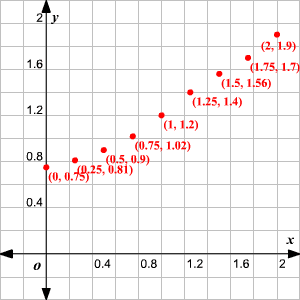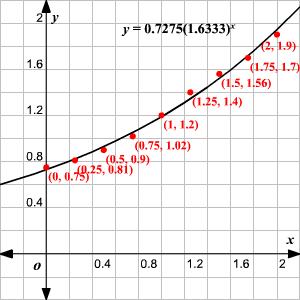# Fitting Equations to Data

Fitting an equation to data is the process of finding a linear, quadratic, exponential, or any other sort of function whose graph includes, or comes as close as possible to, a given set of data in the form of ordered pairs.

The relative predictive power of a model shows how accurately the model is related to the data.

Example :

Plot the set of data points below. Determine whether to use a linear , quadratic , or exponential regression equation. Find the regression equation and graph it.

$\begin{array}{l}\left(0,0.75\right),\left(0.25,0.81\right),\left(0.5,0.9\right),\left(0.75,1.02\right),\left(1,1.2\right)\\ \left(1.25,1.4\right),\left(1.5,1.56\right),\left(1.75,1.7\right),\left(2,1.9\right)\end{array}$

Solution

Enter the $x$ and $y$ -coordinates in your calculator and plot the points.The points seem to approach $x$ -axis asymptotically. So, we assume an exponential model.

Use your calculator to determine the equation of the exponential regression of the points plotted.

The equation of the function is $y=0.7275{\left(1.6333\right)}^{x}$ .

Graph the function with the equation $y=0.7275{\left(1.6333\right)}^{x}$ .